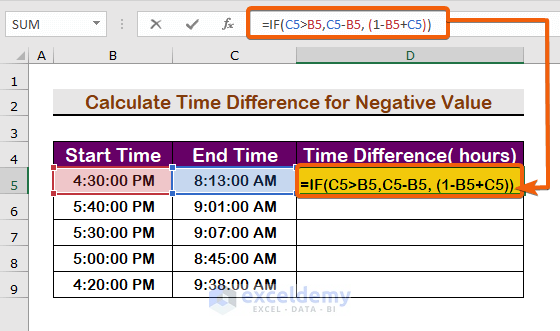# How to Calculate Time Difference Between AM and PM in Excel (6 Ways)

Get FREE Advanced Excel Exercises with Solutions!

There are several functions in Excel that deal with time. However, there is no special function for calculating the difference between two times variables. While you may know, a time value is a numeric value that is represented as time, subtracting one value from another produces a decimal number, which must be structured as time again. In this tutorial, we’ll show you how to calculate the time difference between AM and PM in Excel by applying formulas.

## How to Calculate Time Difference Between AM and PM in Excel: 6 Ways

We’ll go through six different approaches to compute the time difference in the sections below. We estimated the time difference in a variety of units, including hours, minutes, seconds, and also for negative values. A sample Data set is provided to do the task.### 1. Use Formula to Calculate Time Difference Between AM and PM in Hours and Minutes

Follow the instructions below to determine the time difference in hours and minutes.

Step 1:

• To subtract the difference from end time to start time, type the following formula in cell D5.
`=C5-B5`Step 2:

• Press Enter.Step 3:

• AutoFill the rest cells with the same formula and get the final results.Notes.  Look carefully that the difference is shown in AM/PM units. But, if you want to show it just the time difference with hours/minutes, you need to change the format. To change the format follow the procedure.

Step 1:

• Press  Ctrl  1  to open the Format cells dialog box.
• Click on the Time option and select the Format as shown in the image below.
• Finally, click on OK.• Therefore, the time difference will be shown in hh:mm.### 2. Calculate the Time Difference Between AM and PM in Hours in Numbers by Applying Formula

The time difference is usually formatted as the Time format. We can’t calculate the difference in hours in decimal numbers in this format. As a result, you must change the format to the Number format. Follow these easy steps to complete the task.

Step 1:

• Firstly, type the formula in cell D4.
`=(C5-B5)*24`Step 2:

• From the Number tab, select the Number.Step 3:

• Therefore, you will find the hours will be changed to decimal numbers.Step 4:

• Repeat the procedure for all cells in the column.### 3. Apply the INT function to Calculate the Time Difference Between AM and PM in Completed Hours

For counting the time difference in a marginal figure we will apply the INT function with the previous method. Follow the steps outlined below to get it done.

Step 1:

• Firstly, enter the following formula.
`=INT((C5-B5)*24)`Step 2:

• Then, press the Enter.Step 3:

• Apply the AutoFill handle tool to fill up the required cells.### 4. Use a Formula to Calculate the Time Difference Between AM and PM in Minutes

We need to multiply the hour by 1440 to get the time difference in minutes. Follow the steps outlined below to see how we did it.

Step 1:

• To calculate in minutes, write the formula in the formula bar.
`=(C5-B5)*1440`Step 2:

• Then, press Enter to the first result.Step 3:

• Finally, fill up the required cells by applying the same.Notes.  Remember that the tome Format has to be in the Number format.

### 5. Apply Formula to Get Time Difference Between AM and PM in Seconds

Additionally. we have to multiply the hours by 86400 to get the hours minutes. Follow the instructions below to get the answers in the unit of seconds.

Step 1:

• At first, type the formula in cell D5.
`=(C5-B5)*86400`Step 2:

• Then, to see the result press Enter.Step 3:

• Therefore, repeat the steps again to fill up the required cells.### 6. Apply the IF Function to Calculate the Time Difference Between AM and PM for a Negative Value

Importantly, the previous formulas won’t work if the end time is less than the start time. So, we will apply the conditional function IF. Follow the steps to know how to use it.

Step 1:

• First of all, write the following formula.
`=IF(C5>B5,C5-B5, (1-B5+C5))`

##Step 2:

• Secondly, press Enter to see the value.Step 3:

• Finally, fill up the cells you need.Notes.   Adding 1 means to add 24 hours in a decimal point. By adding 1, we convert the negative with a positive interval.

## Conclusion

To recap, I hope this tutorial has given you a solid understanding of how to calculate the time difference between AM and PM in Excel by applying formulas. All of these techniques should be learned and used on your data. Examine the practice book and put your newfound knowledge to use. Because of your vital support, we’re motivated to continue developing classes like these.

Stay with us and keep learning.

## What is ExcelDemy?

ExcelDemy Learn Excel & Excel Solutions Center provides free Excel tutorials, free support , online Excel training and Excel consultancy services for Excel professionals and businesses. Feel free to contact us with your Excel projects.Bhubon Costa

Myself, Bhubon Costa, an engineer, is currently employed with Exceldemy as an Excel & VBA Content Developer. I appreciate solving analytical difficulties, finding practical answers, and, for the most part, I enjoy taking on new tasks. I write articles on Exceldemy about various ways to get out of Microsoft Excel's stuck conditions. My passion is to leave my mark on the world through my work and to have an impact on the community who benefit from it.

We will be happy to hear your thoughtsAdvanced Excel Exercises with Solutions PDF